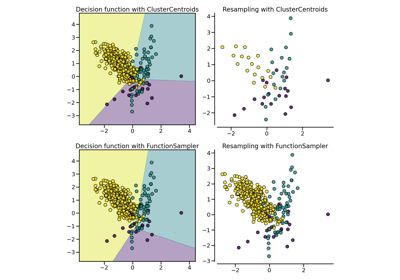# `imblearn.under_sampling`.NeighbourhoodCleaningRule¶

class `imblearn.under_sampling.``NeighbourhoodCleaningRule`(sampling_strategy='auto', return_indices=False, random_state=None, n_neighbors=3, kind_sel='all', threshold_cleaning=0.5, n_jobs=1, ratio=None)[source]

Class performing under-sampling based on the neighbourhood cleaning rule.

Read more in the User Guide.

Parameters
sampling_strategystr, list or callable

Sampling information to sample the data set.

• When `str`, specify the class targeted by the resampling. Note the the number of samples will not be equal in each. Possible choices are:

`'majority'`: resample only the majority class;

`'not minority'`: resample all classes but the minority class;

`'not majority'`: resample all classes but the majority class;

`'all'`: resample all classes;

`'auto'`: equivalent to `'not minority'`.

• When `list`, the list contains the classes targeted by the resampling.

• When callable, function taking `y` and returns a `dict`. The keys correspond to the targeted classes. The values correspond to the desired number of samples for each class.

return_indicesbool, optional (default=False)

Whether or not to return the indices of the samples randomly selected.

Deprecated since version 0.4: `return_indices` is deprecated. Use the attribute `sample_indices_` instead.

random_stateint, RandomState instance or None, optional (default=None)

Control the randomization of the algorithm.

• If int, `random_state` is the seed used by the random number generator;

• If `RandomState` instance, random_state is the random number generator;

• If `None`, the random number generator is the `RandomState` instance used by `np.random`.

Deprecated since version 0.4: `random_state` is deprecated in 0.4 and will be removed in 0.6.

n_neighborsint or object, optional (default=3)

If `int`, size of the neighbourhood to consider to compute the nearest neighbors. If object, an estimator that inherits from `sklearn.neighbors.base.KNeighborsMixin` that will be used to find the nearest-neighbors.

threshold_cleaningfloat, optional (default=0.5)

Threshold used to whether consider a class or not during the cleaning after applying ENN. A class will be considered during cleaning when:

Ci > C x T ,

where Ci and C is the number of samples in the class and the data set, respectively and theta is the threshold.

n_jobsint, optional (default=1)

The number of threads to open if possible.

ratiostr, dict, or callable

Deprecated since version 0.4: Use the parameter `sampling_strategy` instead. It will be removed in 0.6.

Notes

See the original paper: [Re169a8a30019-1].

Supports multi-class resampling. A one-vs.-rest scheme is used when sampling a class as proposed in [Re169a8a30019-1].

References

Re169a8a30019-1(1,2)

J. Laurikkala, “Improving identification of difficult small classes by balancing class distribution,” Springer Berlin Heidelberg, 2001.

Examples

```>>> from collections import Counter
>>> from sklearn.datasets import make_classification
>>> from imblearn.under_sampling import NeighbourhoodCleaningRule
>>> X, y = make_classification(n_classes=2, class_sep=2,
... weights=[0.1, 0.9], n_informative=3, n_redundant=1, flip_y=0,
... n_features=20, n_clusters_per_class=1, n_samples=1000, random_state=10)
>>> print('Original dataset shape %s' % Counter(y))
Original dataset shape Counter({1: 900, 0: 100})
>>> ncr = NeighbourhoodCleaningRule()
>>> X_res, y_res = ncr.fit_resample(X, y)
>>> print('Resampled dataset shape %s' % Counter(y_res))
Resampled dataset shape Counter({1: 877, 0: 100})
```
Attributes
sample_indices_ndarray, shape (n_new_samples)

Indices of the samples selected.

New in version 0.4: `sample_indices_` used instead of `return_indices=True`.

`__init__`(self, sampling_strategy='auto', return_indices=False, random_state=None, n_neighbors=3, kind_sel='all', threshold_cleaning=0.5, n_jobs=1, ratio=None)[source]

Initialize self. See help(type(self)) for accurate signature.

`fit`(self, X, y)[source]

Check inputs and statistics of the sampler.

You should use `fit_resample` in all cases.

Parameters
X{array-like, sparse matrix}, shape (n_samples, n_features)

Data array.

yarray-like, shape (n_samples,)

Target array.

Returns
selfobject

Return the instance itself.

`fit_resample`(self, X, y)[source]

Resample the dataset.

Parameters
X{array-like, sparse matrix}, shape (n_samples, n_features)

Matrix containing the data which have to be sampled.

yarray-like, shape (n_samples,)

Corresponding label for each sample in X.

Returns
X_resampled{array-like, sparse matrix}, shape (n_samples_new, n_features)

The array containing the resampled data.

y_resampledarray-like, shape (n_samples_new,)

The corresponding label of X_resampled.

`fit_sample`(self, X, y)[source]

Resample the dataset.

Parameters
X{array-like, sparse matrix}, shape (n_samples, n_features)

Matrix containing the data which have to be sampled.

yarray-like, shape (n_samples,)

Corresponding label for each sample in X.

Returns
X_resampled{array-like, sparse matrix}, shape (n_samples_new, n_features)

The array containing the resampled data.

y_resampledarray-like, shape (n_samples_new,)

The corresponding label of X_resampled.

`get_params`(self, deep=True)[source]

Get parameters for this estimator.

Parameters
deepboolean, optional

If True, will return the parameters for this estimator and contained subobjects that are estimators.

Returns
paramsmapping of string to any

Parameter names mapped to their values.

`set_params`(self, **params)[source]

Set the parameters of this estimator.

The method works on simple estimators as well as on nested objects (such as pipelines). The latter have parameters of the form `<component>__<parameter>` so that it’s possible to update each component of a nested object.

Returns
self

## Examples using `imblearn.under_sampling.NeighbourhoodCleaningRule`¶